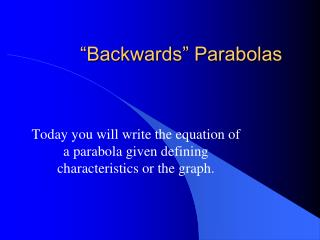DownloadDownload Presentation“Backwards” Parabolas

# “Backwards” Parabolas

Download Presentation## “Backwards” Parabolas

- - - - - - - - - - - - - - - - - - - - - - - - - - - E N D - - - - - - - - - - - - - - - - - - - - - - - - - - -
##### Presentation Transcript

1. “Backwards” Parabolas Today you will write the equation of a parabola given defining characteristics or the graph.

2. Parabolas • Please pick up your clicker. Everything else should be cleared off of your table/desk. • This quiz is to be taken individually. • Good Luck 

3. Point A is called the • Vertex • Focus • Directrix • Axis of Symmetry • Latus Rectum

4. Point D is called the • Vertex • Focus • Directrix • Axis of Symmetry • Latus Rectum

5. The green line is called the • Vertex • Focus • Directrix • Axis of Symmetry • Latus Rectum

6. The yellow line is called the • Vertex • Focus • Directrix • Axis of Symmetry • Latus Rectum

7. The red line is called the • Vertex • Focus • Directrix • Axis of Symmetry • Latus Rectum

8. The distance between A and D and A and the red line is called • a • h • k • p • d • f

9. The length of the green segment is • 2a • 4a • 2p • 4p

10. Which variable would be squared in the equation for the parabola below? • x • y

11. Find the equation of the parabola that satisfies the given conditions. • Vertex is at (-2,3) • Focus is at (1,3)

12. Find the equation of the parabola that satisfies the given conditions. • Focus is (-3, 4) • Directrix has equation x = - 5

13. Find the equation of the parabola that satisfies the given conditions. • Vertex is at (0,0) • Symmetric about the y-axis • Passes through (3,3)

14. Assignment • A 3.2 Sect II & III